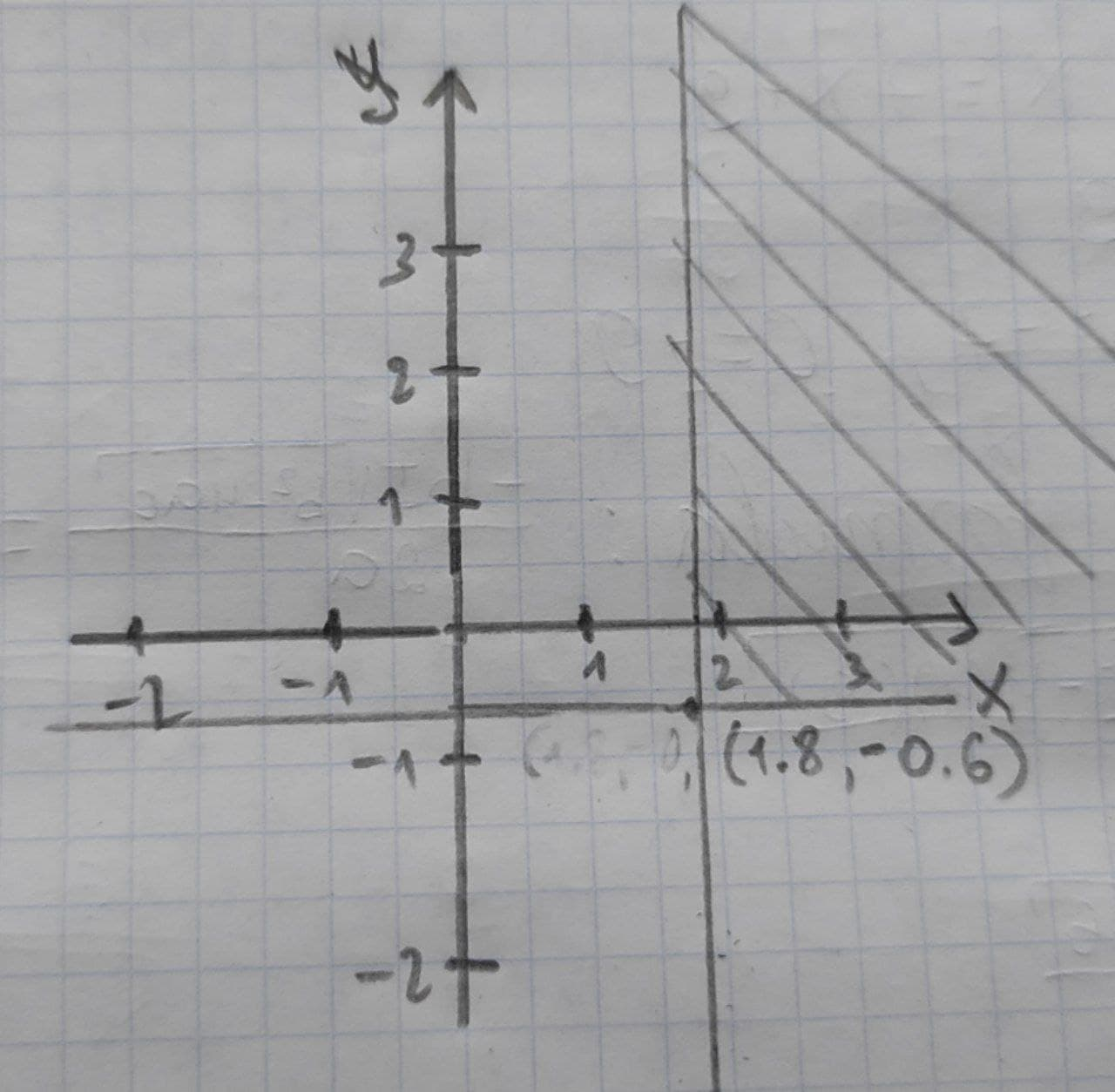# Graph the solution set of the system of inqualities x-2y<3 2x+y>3Tabansi 2020-12-24 Answered
Graph the solution set of the system of inqualities
x-2y<3
2x+y>3
You can still ask an expert for help

## Want to know more about Inequalities systems and graphs?

• Questions are typically answered in as fast as 30 minutes

Solve your problem for the price of one coffee

• Math expert for every subject
• Pay only if we can solve itkrolaniaN

On solving the above inequalities for y, we get
$-2x+4y\succ 6$
$2x+y>3$
$0+5y\succ 3$
$y\succ \frac{3}{5}$
$y\succ 0.6$
On solving the above inequalities for x, we get
$x-2y<3$
$-2x-y<-3$
$-x-3y<0$
$x\succ 3y$
$x\succ 3\left(-0.6\right)\left[\because y\succ 0.6\right]$
$x>1.8$
The solution set of the given inequalities is
$=\left\{\left(x,y\right)\mid X>1.8,y\succ 0.6\right\}$
The graph of the given inequalities is below: